# Hyperbolic partial differential equation

Jump to: navigation, search

at a given point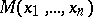A partial differential equation for which the Cauchy problem is uniquely solvable for initial data specified in a neighbourhood ofon any non-characteristic surface (cf. Characteristic surface). In particular, a partial differential equation for which the normal cone has no imaginary zones is a hyperbolic partial differential equation. The differential equation(*)

where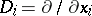(),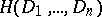is a homogeneous polynomial of degree, while the polynomialis of lower degree than, is a hyperbolic partial differential equation if its characteristic equation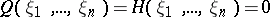hasdifferent real solutions with respect to one of the variables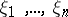, the remaining ones being fixed. Any equation (*) of the first order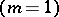with real coefficients is a hyperbolic partial differential equation. A second-order equationis hyperbolic if the quadratic formis positive definite.

#### Comments

The special variable among thesuch that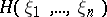hasdifferent real solutions for each set of fixed values of the other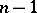is often taken to be(time). One speaks then of a (strictly) hyperbolic equation or an equation of (strictly) hyperbolic type with respect to the-direction. More generally one considers hyperbolicity with respect to a vector[a1].

A polynomialof degreewith principal partis called hyperbolic with respect to the real vectorif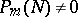and there exists a number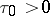such thatIfis such thatand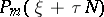has only simple real roots for every real, thenis said to be strictly hyperbolic or hyperbolic in the sense of Petrovskii.

The Cauchy problem for a constant-coefficient differential operatorwith data on a non-characteristic plane is well posed for arbitrary lower-order terms if and only ifis strictly hyperbolic. For a discussion of similar matters for polynomialswith variable coefficients cf. [a2].

For a system of higher-order linear partial differential equations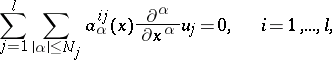where, is a hyperbolic system of partial differential equations in the sense of Petrovskii if the determinant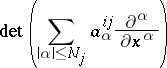calculated in the ring of differential operators is a hyperbolic polynomial in the sense of Petrovskii (as a polynomial of degree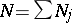). The Cauchy problem for a system that is hyperbolic in this sense is well posed [a3], [a4].

Instead of strictly hyperbolic one also finds the term strongly hyperbolic and instead of hyperbolic also weakly hyperbolic (which is therefore the case in which the lower-order terms ofdo matter).